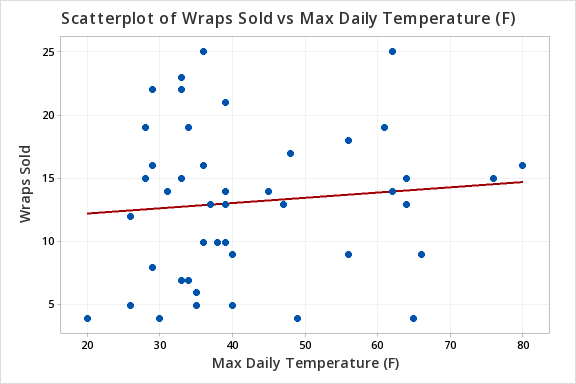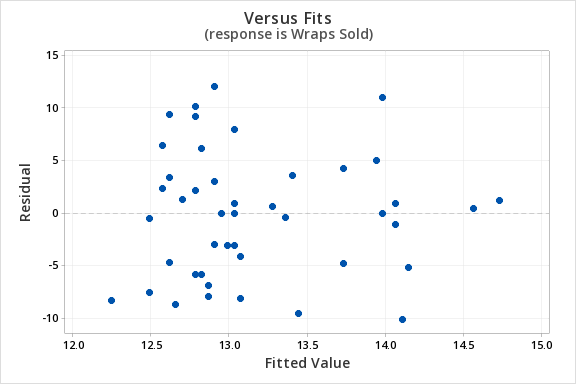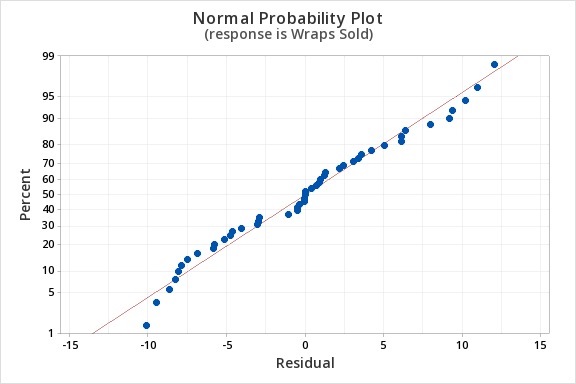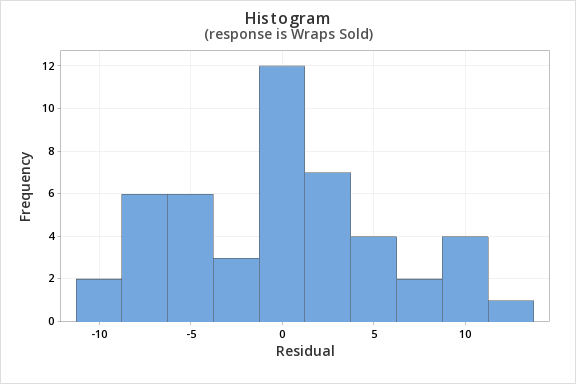# 12.3.4.2 - Example: Business Decisions

A student-run cafe wants to use data to determine how many wraps they should make today. If they make too many wraps they will have waste. But, if they don't make enough wraps they will lose out on potential profit. They have been collecting data concerning their daily sales as well as data concerning the daily temperature. They found that there is a statistically significant relationship between daily temperature and coffee sales. So, the students want to know if a similar relationship exists between daily temperature and wrap sales. The video below will walk you through the process of using simple linear regression to determine if the daily temperature can be used to predict wrap sales.  The screenshots and annotation below the video will walk you through these steps again.

Can daily temperature be used to predict wrap sales?

For the analysis you can use the Minitab file: cafedata.mpx

1. Check assumptions and write hypotheses
• $$H_0\colon \beta_1 =0$$
• $$H_a\colon \beta_1 \neq 0$$

The scatterplot below shows that the relationship between maximum daily temperature and wrap sales is linear (or at least it's not non-linear). Though the relationship appears to be weak.The plot of residuals versus fits below can be used to check the assumptions of independent errors and equal error variances. There is not a significant correlation between the residuals and fits, therefore the assumption of independent errors has been met. The variance of the residuals is relatively consistent for all fitted values, therefore the assumption of equal error variances has been met.Finally, we must check for the normality of errors. We can use the normal probability plot below to check that our data points fall near the line. Or, we can use the histogram of residuals below to check that the errors are approximately normally distributed.Now that we have check all of the assumptions of simple linear regression, we can examine the regression model.

2. Calculate the test statistic
From Minitab...
##### Regression Equation

Wraps Sold = 11.42 + 0.0414 Max Daily Temperature (F)

##### Coefficients
Term Coef SE Coef T-Value P-Value VIF
Constant 11.42 2.66 4.29 0.000
Max Daily Temperature (F) 0.0414 0.0603 0.69 0.496 1.00
##### Model Summary
5.90208 1.04% 0.00% 0.00%
##### Analysis of Variance
Regression 1 16.41 16.41 0.47 0.496
Max Daily Temperature (F) 1 16.41 16.41 0.47 0.496
Error 45 1567.55 34.83
Lack-of-Fit 24 875.17 36.47 1.11 0.411
Pure Error 21 692.38 32.97
Total 46 1583.96

$$t = 0.69$$

3. Determine the p-value

$$p=0.496$$

4. Make a decision

$$p > \alpha$$, fail to reject the null hypothesis

5. State a "real world" conclusion

There is not enough evidence to conclude that maximum daily temperature can be used to predict the number of wraps sold in the population of all days.

  Link ↥ Has Tooltip/Popover Toggleable Visibility